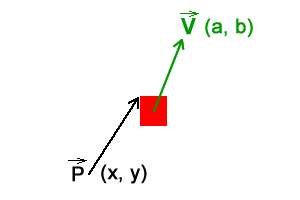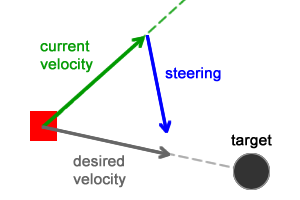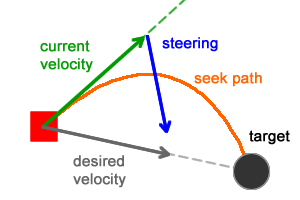# Understanding Steering Behaviors: SeekThis post is part of a series called Understanding Steering Behaviors.
Understanding Steering Behaviors: Flee and Arrival

Steering behaviors aim to help autonomous characters move in a realistic manner, by using simple forces that are combined to produce life-like, improvisational navigation around the characters' environment. In this tutorial I will cover the basic theory behind the seek steering behavior, as well as its implementation.

The ideas behind these behaviors were proposed by Craig W. Reynolds; they are not based on complex strategies involving path planning or global calculations, but instead use local information, such as neighbors' forces. This makes them simple to understand and implement, but still able to produce very complex movement patterns.

Note: Although this tutorial is written using AS3 and Flash, you should be able to use the same techniques and concepts in almost any game development environment. You must have a basic understanding of math vectors.

## Position, Velocity and Movement

The implementation of all forces involved in steering behaviors can be achieved using math vectors. Since those forces will influence the character's velocity and position, it's a good approach to use vectors to represent them as well.

Even though a vector has a direction, it will be ignored when related to position (let's assume the position vector is pointing to the character's current location).The figure above represents a character positioned at (x, y) with a velocity (a, b). The movement is calculated using  Euler integration:

 1 position = position + velocity 

The direction of the velocity vector will control where the character is heading to while its length (or magnitude) will control how much it will move every frame. The greater the length, the faster the character moves. The velocity vector can be truncated to ensure it will not be greater than a certain value, usually max velocity. Below is a test showing that approach.

Move the mouse to move the target.

The red square is moving towards a target (the mouse cursor). This movement pattern illustrates the seek behavior without any steering forces being applied so far. The green line represents the velocity vector, calculated as follows:

 1 velocity = normalize(target - position) * max_velocity 

It's important to notice that without the steering force, the character describes straight routes and it instantly changes its direction when the target moves, thus making an abrupt transition between the current route and the new one.

## Calculating Forces

If there was only the velocity force involved, the character would follow a straight line defined by the direction of that vector. One of the ideas of steering behaviors is to influence the character's movement by adding forces (called steering forces). Depending on those forces, the character will move in one or another direction.

For the seek behavior, the addition of steering forces to the character every frame makes it smoothly adjust its velocity, avoiding sudden route changes. If the target moves, the character will gradually change its velocity vector, trying to reach the target at its new location.

The seek behavior involves two forces: desired velocity and steering:The desired velocity is a force that guides the character towards its target using the shortest path possible (straight line between them - previously, this was the only force acting on the character). The steering force is the result of the desired velocity subtracted by the current velocity and it pushes the character towards the target as well.

Those forces are calculated as follows:

 1 desired_velocity = normalize(target - position) * max_velocity  2 steering = desired_velocity - velocity 

After the steering force has been calculated, it must be added to the character (it will be added to the velocity force). The addition of the steering  force to the velocity every frame will make the character smoothly abandon its old straight route and head towards the target, describing a seek path (orange curve in the figure below):The addition of those forces and the final velocity/position calculation are:

 1 steering = truncate (steering, max_force)  2 steering = steering / mass  3 4 velocity = truncate (velocity + steering , max_speed)  5 position = position + velocity 

The steering force is truncated to ensure it will not exceed the amount of allowed forces the character can handle. Also the steering force is divided by the character mass, which produces different movement speeds for different weighted characters. Below is a test showing the seek behavior with all forces applied:

Move the mouse to move the target.

Every time the target moves, each character's desired velocity vector changes accordingly. The velocity vector, however, takes some time to change and start pointing at the target again. The result is a smooth movement transition.

## Conclusion

Steering behaviors are great for creating realistic movement patterns. The main idea is to use local information to calculate and apply forces to create the behaviors. Even though the calculation is simple to implement, it is still able to produce very complex results.

This tutorial described the basics of steering behaviors, explaining the seek behavior. Over the next few posts, we will learn about more behaviors. Check out the next post: Flee and Arrival.